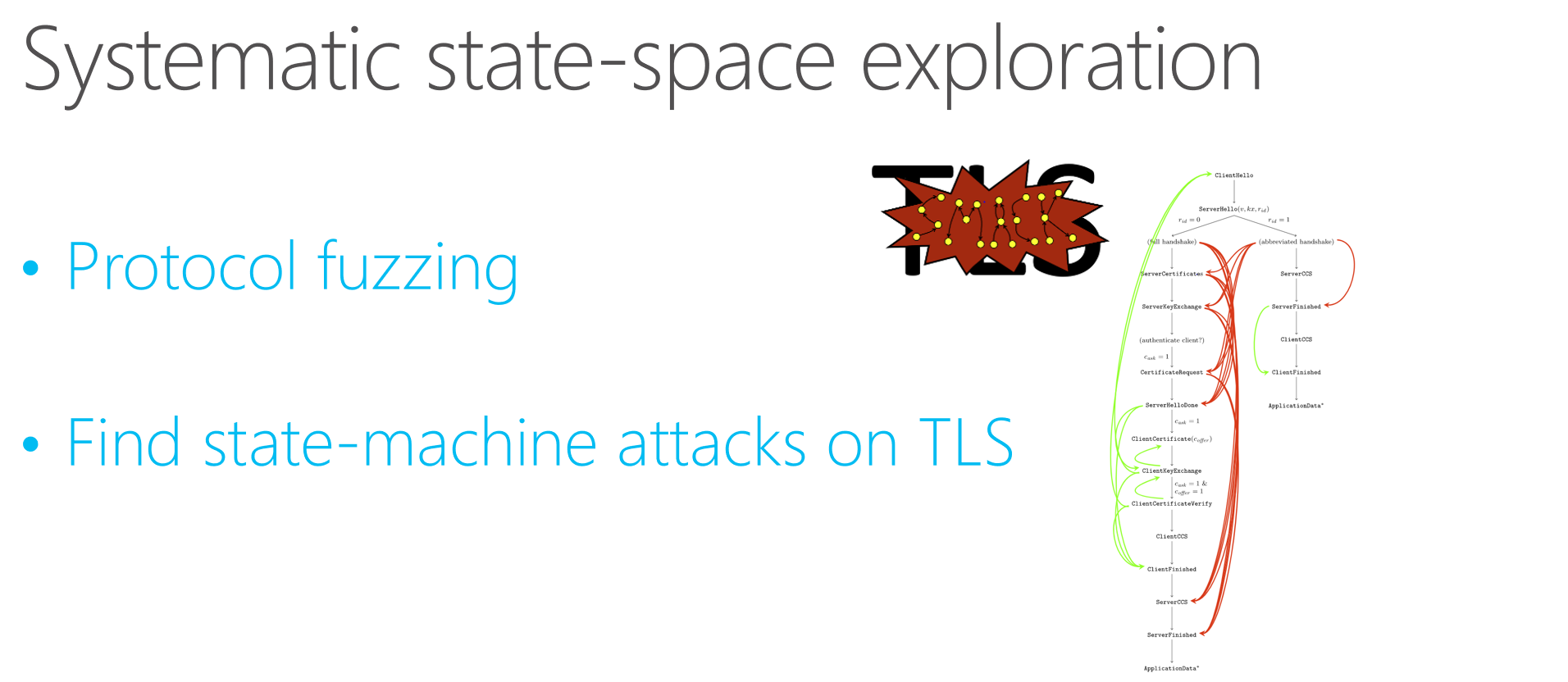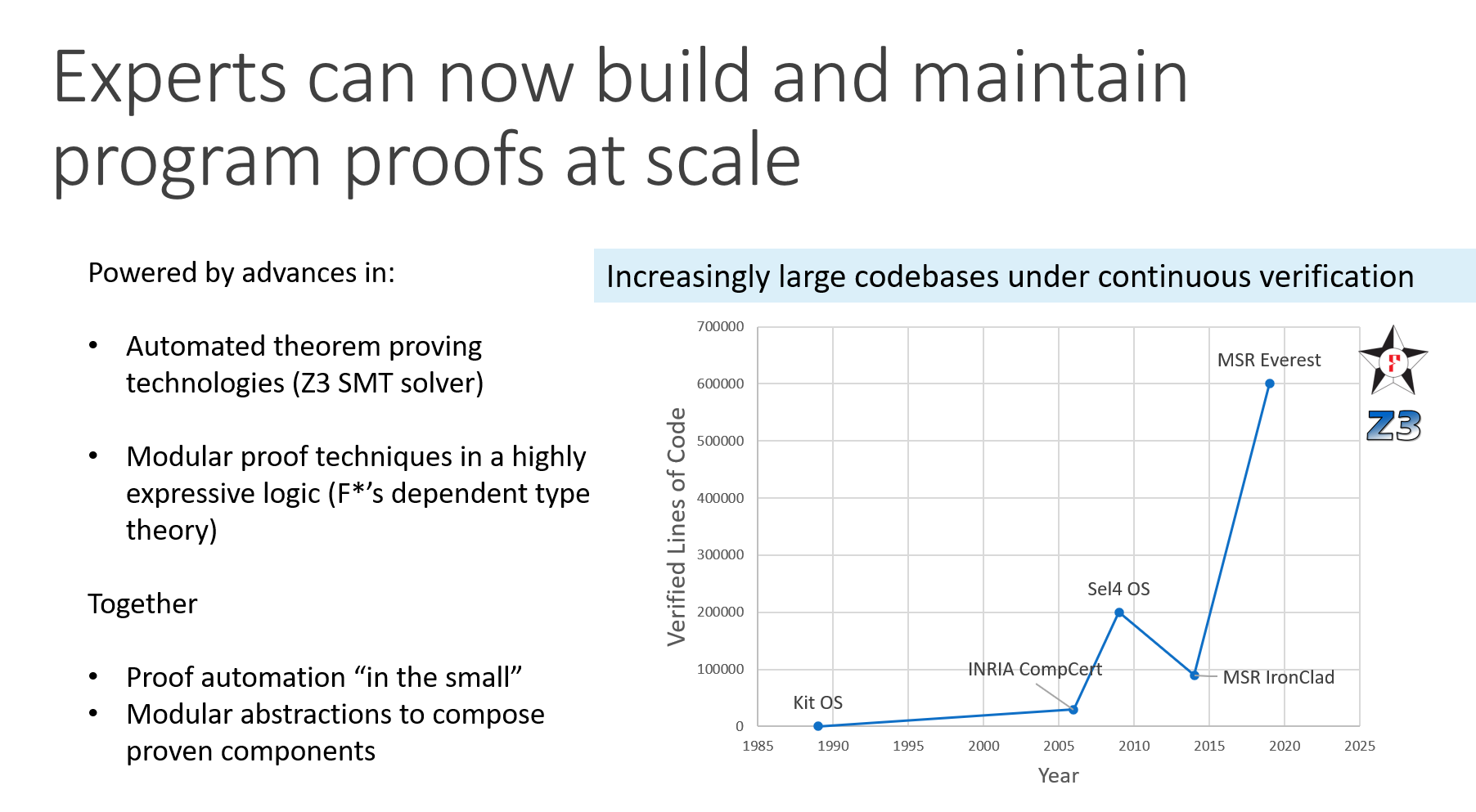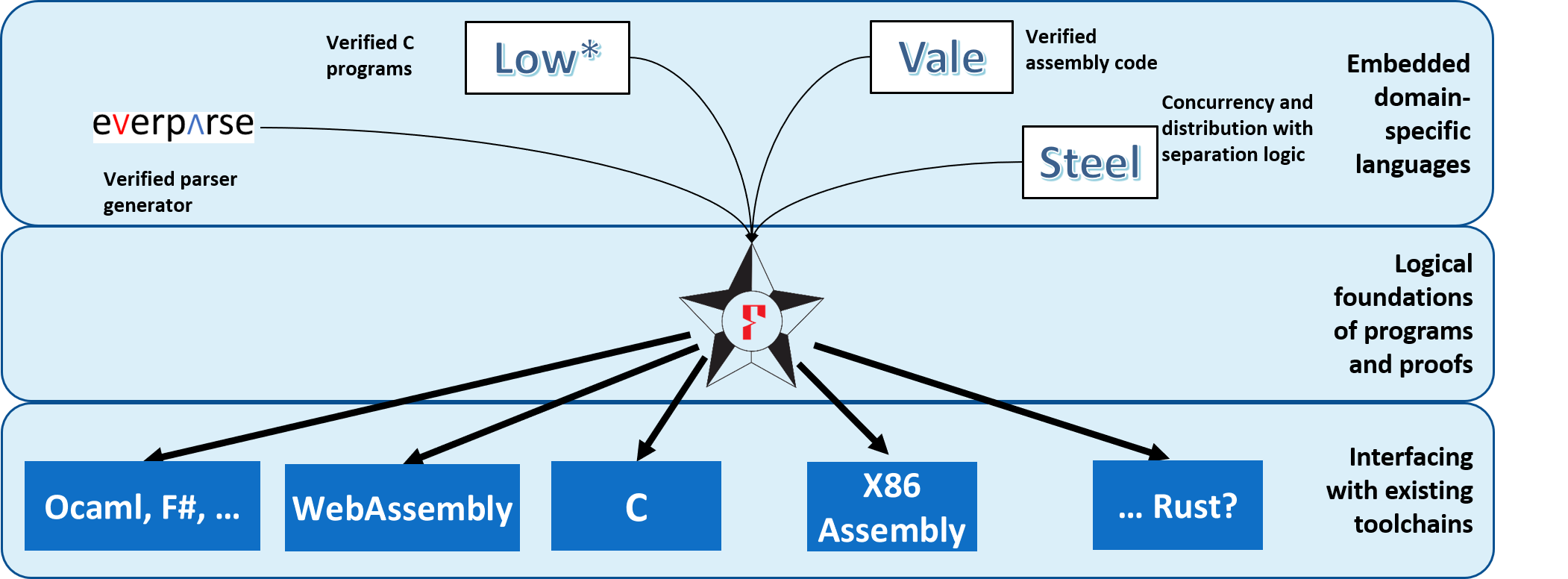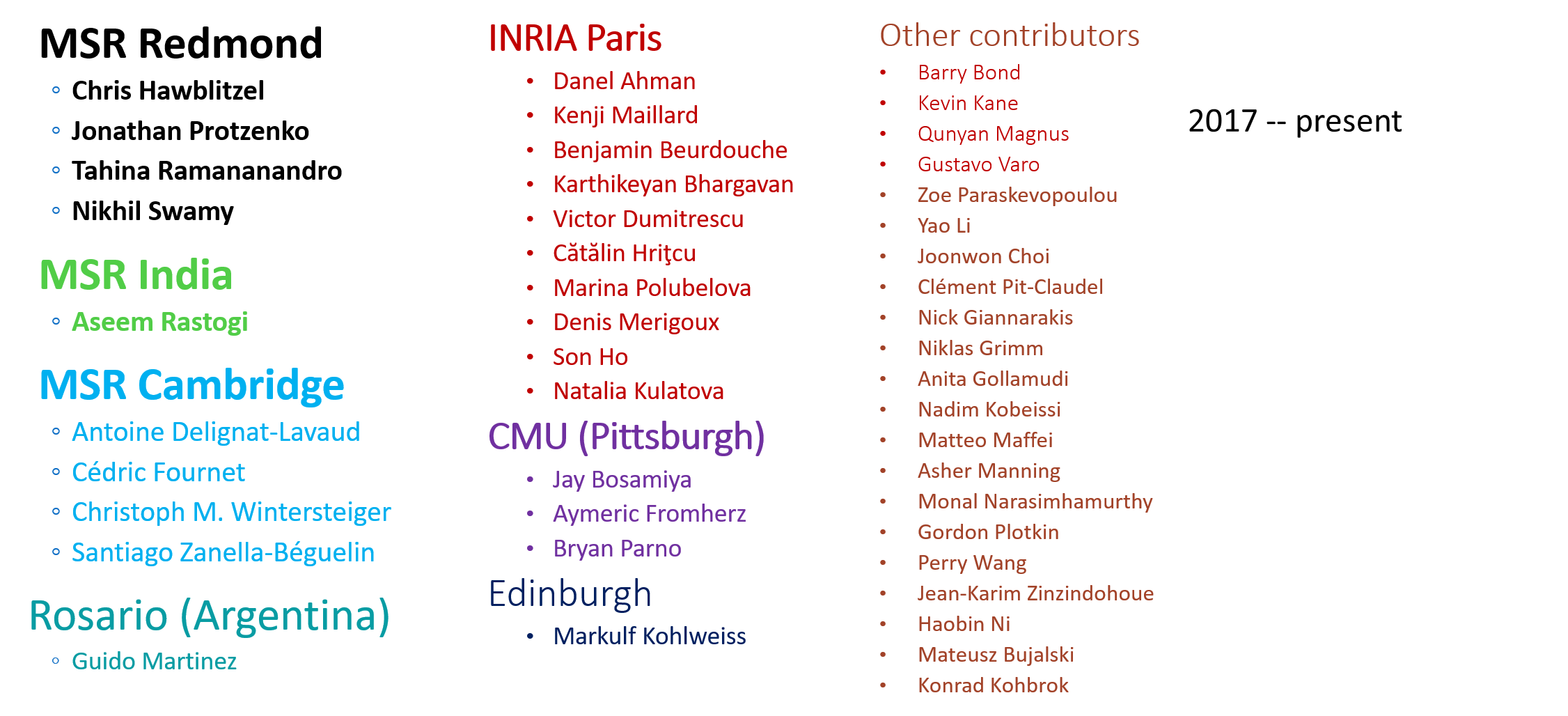## Proof-oriented Programming in F*

Microsoft Research

Goldman Sachs Seminar, March 10, 2021

## Through Code Analysis (1)## Through Code Analysis (2)## Through Code Analysis (3)### Full-scale Program Proofs

• Mathematical specifications of correctness and security
• Machine-checked proof that the code does not deviate from the spec
• Foundational: Against a formal machine model
• Integrated: Single theorem covering all the code### What is F*?

• Functional programming language with effects

• F* extracted to OCaml or F# by default
• Subset of F* compiled to efficient C code and to Wasm
• Semi-automated program verifier using automated theorem proving

• like Dafny, FramaC, Why3,
• With an expressive core language based on dependent type theory

• like Coq, Lean, Agda, Idris,
• A metaprogramming and tactic framework for interactive proof and user-defined automation

• like Coq, Isabelle, Lean, PVS, etc.
• And many foundational program logics for embedded DSLs

• Many variants of Hoare logic for sequential programs
• Concurrent separation logic, for concurrent and distributed programs
• Relational Hoare logic, for program equivalence and security proofs

### A first taste

• Write code in a syntax similar to OCaml, F#, Standard ML:

let rec factorial n =
if n = 0 then 1
else n * factorial (n - 1)
• Give it a specification, claiming that factorial is a total function from non-negative to positive integers.

val factorial: n:int{n >= 0} -> Tot (i:int{i >= 1})
• Ask F* to check it

fstar factorial.fst
Verified module: Factorial
All verification conditions discharged successfully

## The F* Landscape## Program Proofs in F* for Billions of Unsuspecting Users## Contributors## Contributors

Microsoft Research, Inria Paris, CMU, MIT, Rosario, …

• many former members, interns, external contributors, you?

### The rest of this lecture

#### Today

• The functional core of F*

• Several style of proof illustrated on simple functional programs

• Encoding effects: Simple verified stateful programming

• Advanced stuff: A taste of what is possible

### Basic types

• Informal mental model: Types as sets of values

• The empty type: It has no values

type empty =
• The singleton: It has exactly 1 value

type unit = ()
• Boolean: It has exactly 2 values

type bool = true | false

### The functional core of F*

• Recursive functions

let rec factorial (n:int) : int =
if n = 0 then 1 else n * (factorial (n - 1))
• Inductive datatypes (immutable) and pattern matching

type list (a:Type) =
| []     (* Nil  *) : list a
| _ :: _ (* Cons *) : hd:a -> tl:list a -> list a

let rec map (f: a -> b) (x:list a) : list b =
match x with
| [] -> []
| hd :: tl -> f hd :: map f tl
• Lambdas (unnamed, first-class functions)

map (fun x -> x + 42) [1;2;3] ~> [43;44;45]

### Refinement types

type nat = x:int{x>=0}
• Informal mental model: A type describes a set of values

let empty = x:int { false } //one type for the empty set
let zero = x:int{ x = 0 } //the type containing one element 0
let pos = x:int { x > 0 } //the positive numbers
let neg = x:int { x < 0 } //the negative numbers
let even = x:int { x % 2 = 0 } //the even numbers
let odd = x:int { x % 2 = 1 } //the odd numbers
let prime = x:nat { forall n. x % n = 0 ==> n = 1 || n = x } //prime numbers
• Refinements introduced by type annotations (code unchanged)

let rec factorial (n:nat) : nat = if n = 0 then 1 else n * (factorial (n - 1))
• Logical obligations discharged by SMT (simplified)

n >= 0, n <> 0 |= n - 1 >= 0
n >= 0, n <> 0, factorial (n - 1) >= 0 |= n * (factorial (n - 1)) >= 0
• Refinements eliminated by subtyping: nat<:int

let i : int = factorial 42
let f : x:nat{x>0} -> int = factorial

### Dependent types

• Dependent function types (), here together with refinements:

val incr : x:int -> y:int{x < y}
let incr x = x + 1
• Can express pre- and post- conditions of pure functions

val incr : x:int -> y:int{y = x + 1}
• Exercise: Can you find other types for incr?

### Total functions in F*

• The F* functions we saw so far were all total

• Tot effect (default) = no side-effects, terminates on all inputs

val factorial : nat -> Tot nat
let rec factorial n = (if n = 0 then 1 else n * (factorial (n - 1)))
• Quiz: How about giving this weak type to factorial?

val factorial : int -> Tot int
  let rec factorial n = (if n = 0 then 1 else n * (factorial (n - 1)))
^^^^^
Subtyping check failed; expected type (x:int{x << n}); got type int

factorial (-1) loops! (int type in F* is unbounded)

### Semantic termination checking

• based on well-founded ordering on expressions (<<)
• naturals related by < (negative integers unrelated)
• inductives related by subterm ordering
• lex tuples %[a;b;c] with lexicographic ordering
• order constraints discharged by the SMT solver
• arbitrary total expression as decreases metric
val ackermann: m:nat -> n:nat -> Tot nat (decreases %[m;n])
let rec ackermann m n =
if m=0 then n + 1
else if n = 0 then ackermann (m - 1) 1
else ackermann (m - 1) (ackermann m (n - 1))
• default metric is lex ordering of all (non-function) args
val ackermann: m:nat -> n:nat -> Tot nat

## Types are specifications; Total functions are proofs; Recursion is induction

let rec length (xs:list a) : nat =
match xs with
| [] -> 0
| _::tl -> 1 + length tl

let rec append (xs ys : list a) : list a =
match xs with
| [] -> ys
| x :: xs' -> x :: append xs' ys
• Prove that the length of append is the sum of the lengths of its arguments
let rec append_length (xs ys : list a) : Lemma (length (append xs ys) = length xs + length ys)
= match xs with
| [] -> ()
| x :: xs' -> append_length xs' ys
• Proof by induction on xs
• Base case, xs=[] is easy: append [] ys = ys /\ length [] = 0
• Step: Use IH by calling function recursively on smaller arguments
• Sugar: Lemma p = u:unit { p } 

## More Lemmas

let snoc l h = l @ [h]

let rec reverse (l:list a) : list a =
match l with
| [] -> []
| hd::tl -> snoc (reverse tl) hd
let rec rev_snoc (l:list a) (h:a)
: Lemma (reverse (snoc l h) == h::reverse l)
= match l with
| [] -> ()
| hd::tl -> rev_snoc tl h
let rec rev_involutive (l:list a)
: Lemma (reverse (reverse l) == l)
= match l with
| [] -> ()
| hd::tl -> rev_involutive tl; rev_snoc (reverse tl) hd

## Proof of a program: QUICKSORT

let rec quicksort (f:total_order a) (l:list a)
: Tot (m:list a{sorted f m /\ is_permutation l m})
(decreases (length l))
= match l with
| [] -> []
| pivot::tl ->
let hi, lo = partition (f pivot) tl in
let m = quicksort f lo @ pivot :: quicksort f hi in
permutation_app_lemma pivot tl (quicksort f lo) (quicksort f hi);
m
let permutation_app_lemma (hd:a) (tl:list a)
(l1:list a) (l2:list a)
: Lemma (requires (is_permutation tl (l1 @ l2)))
(ensures (is_permutation (hd::tl) (l1 @ (hd::l2))))

## Beyond refinements: Indexed types

• Length-indexed vectors

type vec a : nat -> Type =
| Nil : vec a 0
| Cons : hd:a -> tl:vec a n -> vec a (n + 1)

let rec append (v1:vec a n) (v2:vec a m)
: vec a (n + m) =
match v1 with
| Nil -> v2
| Cons hd tl -> Cons hd (append tl v2)
• Red-Black Trees

type rbtree a : nat -> color -> Type =
| Leaf : rbnode a 1 Black
| R    : left:rbnode a h Black -> value:a -> right:rbnode a h Black -> rbnode a h Red
| B    : left:rbnode a h cl -> value:a -> right:rbnode a h cr -> rbnode a (h+1) Black
• Concurrent computations indexed by separation logic specs

type m : a:Type -> p:slprop -> q:(a -> slprop) -> Type =
| Ret: post:post_t st a -> x:a -> m st a (post x) post
| Bind: ...
| Act: ...
| Frame: ...
| Par: ...

### Values and Computations

• “Values” aka unconditionally total terms

• Two classes of types

• Value types (t): int, list int,
• Computation types (C): conditionally pure, divergent, stateful,
• Dependent function types of the form: x:t -> C

• F* is call-by-value
• argument can't have side-effects, so value type
• Two forms of refinement types

• Refined value types: x:t{p}
• Refined computation types:
• Stateful computations that can read and write the heap: ST t pre post

### The divergence effect (Dv)

• Some useful code really is not always terminating, e.g., an interpreter for a Turing complete language

let rec eval (e:exp) : Dv exp =
match e with
| App (Lam x e1) e2 -> eval (subst x e2 e1)
| App e1 e2 -> eval (App (eval e1) e2)
| Lam x e1 -> Lam x (eval e1)
| _ -> e
(* (\x. x x) (\x. x x) *)
let main = eval (App (Lam 0 (App (Var 0) (Var 0)))
(Lam 0 (App (Var 0) (Var 0))))
  let rec server () =
let x = get_request () in
fork (handle x);
server()

### F* effect system encapsulates effectful code

• Pure code cannot call potentially divergent code

• Only pure code can appear in specifications

val eval : expr -> Dv expr
    type tau = e:expr{e = eval e'}
^^^^^^
Expected a pure expression; got an expression ... with effect "DIV"
• Sub-effecting: Tot t <: Dv t
(e.g. divergent code can include pure code)

val subst : list (var * expr) -> expr -> Tot expr
eval (subst [x, Num 0] e) : Dv expr

### Verifying potentially divergent programs

#### (partial correctness)

• Using refinement types
val factorial : nat -> Dv nat
• Or the Div computation type (pre- and post- conditions)
let rec eval_closed (e:exp) : Div exp
(requires closed e)
(ensures fun e' -> Lam? e' /\ closed e')
= match e with
| App e1 e2 -> let Lam e1' = eval_closed e1 in
below_subst_beta 0 e1' e2;
eval_closed (subst (sub_beta e2) e1')
| Lam e1 -> Lam e1
• Dv just an abbreviation
Dv t = Div t (requires True) (ensures (fun _ -> True))

type st (a:Type) = heap -> Tot (a * heap)
new_effect {
STATE : a:Type -> Effect
with repr = st;
return = fun (a:Type) (x:a) (h:heap) -> x, h;
bind = fun (a b:Type) (f:st a) (g:a -> st b) (h:heap) ->
let z, h' = f h in g z h';
get = fun () (h:heap) -> h,h;
put = fun (h:heap) _ -> (),h
}
• this monadic definition is the model F* uses to verify stateful code
• It is an executable model, and you can choose to run your programs in the model
• But, state can be primitively implemented under the hood if you like
• for instance by C stack+heap

### General approach to effectful programming

• Program libraries to model effects

• Derive effectful actions for primitive operations

• read, write, alloc, throw, catch, fork, join, etc.
• Write programs against these models and verify them with refined computation types

• Extract them to OCaml, F#, C, Wasm, Java? with primitive effects

• F*:

let incr (r:ref int) : ST unit
(ensures fun h0 _ h1 -> modifies {r} h0 h1 /\ h1.[r] = h0.[r] + 1)
= r := !r + 1

ML:

let incr (r:ref int) : unit = r := !r + 1

C:

void incr (int *r) { *r = *r + 1; }`

### F* as a Verification Backend

• But, you need to work quite hard to tune the verifier to

• Get predictable and fast proofs
• Translate failed verifications back to the user
• Encoding Java in F*, not likely to be easy

• Identify a small fragment of Java
• Or consider going the other direction: generate Java from verified F*, e.g., via Scala?